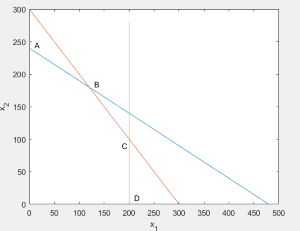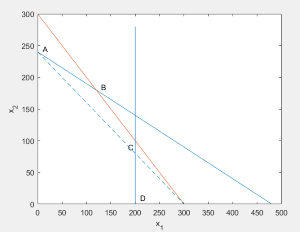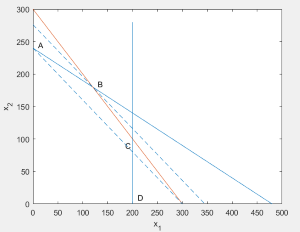# 简单易懂的单纯形法理解

### 简单易懂的单纯形法理解

$\qquad$从学线性规划开始一直觉得单纯形是一种奇奇怪怪不知所云的方法 （居然还好意思叫simplex，我觉得叫complex才对）， 上课老师讲到的一大堆性质定理的证明也让人晕头转向，最后还是死记硬背下了单纯形表的解法勉强应付过了作业。直到今天小班课助教讲了他学到的对于单纯形的理解，才觉得豁然开朗，原来单纯形的确是这么“单纯”。和图解法结合起来也更加清晰直观地看到了单纯形法的工作过程，分享出来希望也能够帮助大家更好地理解。我们不需要任何定理公式

$\qquad$需要读者关于线性规划标准形的一些最基本的概念，如基变量，基本可行解等，如果不了解可先学习一下标准形，在此不对概念做赘述。以及知道单纯形的大概思路（先确定一组基本可行解，然后通过各种操作使得目标函数的值不断下降，最终得到最优解

$\qquad$我们以《算法设计与分析（第2版）》上的例子来进行讲解

$max \qquad z=12x+15y\\ s.t.\quad 0.25x+0.50y\ \leq120\\ \qquad \;0.50x+0.50y\ \leq150\\\qquad0.25x\qquad\qquad\leq50\\x\geq0,\quad y\geq0$$\qquad$这是最简单的情形，所有的约束条件都为小于等于，我们可以直接通过引入松弛变量来化为标准形，即：
$min \qquad -z=-12x_{1}-15x_{2}\\ s.t.\quad 0.25x_{1}+0.50x_{2}+x_{3}\ =120\\ \qquad \;0.50x_{1}+0.50x_{2}+x_{4}\ =150\\\qquad0.25x_{1}\qquad\quad\quad\;+x_{5}=50\\x_{j}\geq0,\quad j=1,2,...,5$

$\qquad$单纯形的第一步是找出一组初始可行基，对于我们题目中这种约束条件全为小于等于的版本，我们只需取三个松弛变量作为初始可行基即可。

$x_{B}$ $b$ $x_{1}\qquad\qquad x_{2}\qquad\qquad x_{3}\qquad\qquad x_{4}\qquad\qquad x_{5}$ $\theta$
$x_{3}$ 120 $0.25 \qquad \qquad0.50\qquad\qquad1\qquad\qquad0\qquad\qquad0\quad$ 240
$x_{4}$ 150 $0.50 \qquad \qquad0.50\qquad\qquad0\qquad\qquad1\qquad\qquad0\quad$ 300
$x_{5}$ 50 $0.25 \qquad \qquad0\qquad\qquad\quad0\qquad\qquad0\qquad\qquad1\quad$
$-z$ 0 $-12 \qquad \ \ \quad-15\ \ \qquad\qquad0\qquad\qquad0\qquad\qquad0\quad$

$\qquad$让我们首先理解这个单纯形表表示的是什么意思。先抛开$\ \theta\;$列不看，只看前面的几列。$x_{B}$列表示的是我们当前选取的基变量，而$\;b\;$列和$\;x\;$列合起来就是我们目前线性规划的约束条件，最下面一行是我们当前的目标函数，由于我们目前选择的初始可行解为{0,0,120,150,50}，所以初始目标函数值为0。相信到这里大家都是能够理解的。

$\qquad$接下来就开始我们的优化，我们的目的是想让目标函数的值降低，而现在影响目标函数的变量有$x_{1} 和 x_{2}$两个变量增大均能够使得目标函数减小，我们先选择其中的一个来达成我们的第一步。注意到，$x_{1}$每增加1会使得$\;-z\;$减小12，而$x_{2}$每增加1会使得$\;-z\;$减小15。我们希望目标函数减小的越快越好，所以这里我们选择$x_{2}$进行增大，用单纯形的术语来说就是让$x_{2}$作为换入变量，使它成为可行基。

$\qquad$我们当然希望目标函数越小越好，那么我们的$x_{2}$自然是越大越好，但是它不能无限制地增大下去，因为我们有约束条件（三个等式，以及非负条件）。那么我们考察一下$x_{2}$最多能增大多少呢？含有$x_{2}$的等式限制是前两个：$\qquad 0.25x_{1}+0.50x_{2}+x_{3}\ =120\\ \qquad \;0.50x_{1}+0.50x_{2}+x_{4}\ =150$由于它们是等式条件，所以如果想要$x_{2}$增大，必须有相应变量减小来维持等式条件成立，由于非负条件的限制，两式中能减小的只能分别是$x_{3}$$x_{4}$了（$x_{1}=0$）。
$\qquad$现在$x_{3}$$x_{4}$的值均为1，要让$x_{2}$增加到最大就要让它们减小到0。让$x_{3}$减小到0可以使$x_{2}$增大到$1*120/0.50=240$，而让$x_{4}$减小到0可以使$x_{2}$增大到$1*150/0.50=300$。有没有发现，这就是我们的$\;\theta\;$那一列的值？注意这里有一个比较容易迷惑的点。 有些同学可能会想，我们想让$x_{2}$越大越好，那这里300更大是不是能增大到300？这样思考是不对的。要知道，这两个等式是约束条件，是需要都满足的，如果我们选取了300那么第一个等式就不能满足了。从这个角度思考，这里我们其实应该选择的是$\;\theta\;$的最小值，也即让$x_{3}$减小到0，用单纯形的术语来讲就是选择$x_{3}$作为换出变量

$\qquad$现在，我们尽管没有提到变量替换这样的概念，但我们已经选好了所谓的换入变量换出变量。这时候，我们的解向量变成了{0,240,0,1,0}，并且$-z$的值变成了$-15*240=-3600$（根据之前说的$x_{2}$每增大1就会让$-z$减小15）。因为$x_{2}$此时已经不能再增加了，我们也在约束条件中想办法把它的影响减到最小，即只保留用到的等式约束中的$x_{2}$并把它单位化，把其余的等式约束中消去$x_{2}$。这一步通过行列变换可以很容易得到，化简后的等式约束变为：
$\quad\; 0.50x_{1}+x_{2}+2x_{3}\ =240\\ \quad0.50x_{1}-x_{3}+x_{4}\ =30\\\qquad\;0.25x_{1}\qquad\quad\quad\;+x_{5}=50$

$\qquad$到这里，有些同学可能会提出疑问：为什么$x_{2}$$x_{4}$$x_{5}$的系数是0呢？或者是：为什么我们考虑$x_{1}$增大时只考虑了对$x_{2}$的影响而没有考虑对$x_{3}$$x_{4}$$x_{5}$的影响呢？这就涉及到我们对于换出的理解了。事实上，从一开始的目标函数中，$x_{1}$的系数-12就不只是增大1个单位$x_{1}$的影响，而是增大1个$x_{1}$，减小0.25个$x_{3}$，减小0.5个$x_{4}$，减小0.25个$x_{5}$收益，这才是我们系数的真正含义，其他变量的系数也是同理。当我们进行完第一步的单纯形变换后，由于我们的消元，后两个等式中都没有$x_{2}$，故换入换出的比例关系不受到影响，所以我们只需要考虑第一个等式中的关系。同时，我们系数的含义是“增加1单位的$x_{j}$,同时加上对其他变量的影响，一共对目标函数结果的影响”，而$x_{2}$此时已经不能再增大了，自然也就没有系数了。这样我们就完全解释了所有系数的来历，也体会出了我们行列变换消元的重要性所在。

$x_{B}$ $b$ $x_{1}\qquad\qquad x_{2}\qquad\qquad x_{3}\qquad\qquad x_{4}\qquad\qquad x_{5}$ $\theta$
$x_{2}$ 240 $0.50 \qquad \qquad1\qquad\qquad2\qquad\qquad0\qquad\qquad0\quad$ 480
$x_{4}$ 30 $0.25 \qquad \qquad0\quad\qquad-1\qquad\qquad1\qquad\qquad0\quad$ 120
$x_{5}$ 50 $0.25 \qquad \qquad0\quad\qquad\quad0\qquad\qquad0\qquad\qquad1\quad$ 200
$-z$ 3600 $-4.5 \qquad \ \ \quad0\ \ \qquad\qquad30\qquad\qquad0\qquad\qquad0\quad$$\qquad$从图上我们显然可以看到这并不是最优解，最优解应当经过B点，即让直线向右移，即让$x_{1}$增大，那么我们从单纯形表中是不是也能得出同样的结论呢？让我们一起继续来看。

$\qquad$有了第一次单纯形变换的经验，我们很容易同样地进行第二步。首先选择能让目标函数减小的变量，这里只有$x_{1}$可以了，即为换入变量。然后算出$x_{1}$在约束的情况下能变化多少，即$b$列除以$x_{1}$列算出$\;\theta\;$，选取其中最小的一个（必要的约束条件），这里是第二行120，则第二行对应的$x_{B}$$x_{4}$换出变量。然后我们对于$x_{1}$列，把第二个等式单位化，第一三个等式对应行和下面的目标函数行列变换消元，得到新的单纯形表：

$x_{B}$ $b$ $x_{1}\qquad\qquad x_{2}\qquad\qquad x_{3}\qquad\qquad x_{4}\qquad\qquad x_{5}$ $\theta$
$x_{2}$ 240 $0 \qquad \qquad1\qquad\qquad4\qquad\qquad-2\qquad\qquad0\quad$
$x_{1}$ 30 $1 \qquad \qquad0\quad\qquad\quad-4\qquad\qquad4\qquad\qquad0\quad$
$x_{5}$ 50 $0 \qquad \qquad0\quad\qquad\quad1\qquad\qquad-1\qquad\qquad1\quad$
$-z$ 4140 $0 \qquad \ \ \quad0\ \ \qquad\qquad12\qquad\qquad18\qquad\qquad0\quad$©️2019 CSDN 皮肤主题: 大白 设计师: CSDN官方博客